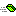## IObject

This is the base interface to allow objects to be utilized throughout most of VBCorLib.

### Public:

#### Methods:

NameDescriptionEquals Determines whether the specified value is equal to the current IObject.GetHashCode Serves as a hash function for a particular type.ToString Returns a string representation of the current object.

#### Remarks

The IObject interface is a low-level interface utilized by VBCorLib to provide basic services when interacting with objects. Many of the classes in VBCorLib implement this interface. By implementing this interface in new classes, those classes can be better serviced by the VBCorLib eco-system.

If this interface is implemented, then the same methods should be implemented in the normal public interface to maintain consistency.

If a class is not expected to be used by VBCorLib, then implementing this interface is not necessary.

#### Examples

The following code example shows a common default implementation of the IObject interface. It simply forwards calls to default method implementations through the MyBase global property.

Override the individual methods for custom functionality.

```Implements IObject

Public Function Equals(ByRef Value As Variant) As Boolean
Equals = MyBase.Equals(Me, Value)
End Function

Public Function GetHashCode() As Long
GetHashCode = MyBase.GetHashCode(Me)
End Function

Public Function ToString() As String
ToString = MyBase.ToString(Me, App)
End Function

'''''''''''''''''''''''''''''''''''''''''''''''''''''''''''''
' Object Interface
'''''''''''''''''''''''''''''''''''''''''''''''''''''''''''''
Private Function IObject_Equals(Value As Variant) As Boolean
IObject_Equals = Equals(Value)
End Function

Private Function IObject_GetHashCode() As Long
IObject_GetHashCode = GetHashCode
End Function

Private Function IObject_ToString() As String
IObject_ToString = ToString
End Function
```